Maharashtra State BoardSSC (English Medium) 7th Standard

# Drawing a double Bar Graph:

1) The number of boys and girls in a school is given. Draw a double bar graph to show this information.

 Class 5th 6th 7th 8th 9th 10th Boys 52 68 67 50 62 60 Girls 57 63 64 48 62 64

### Solution:

Steps for drawing a Joint Bar Graph:

1. On a graph paper, draw the X-axis and Y-axis and their point of intersection.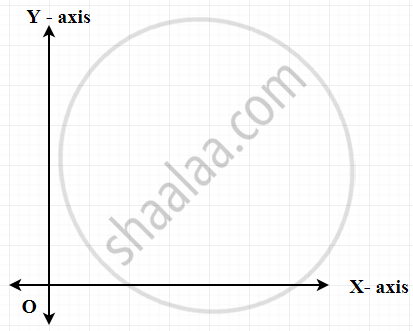2. Keeping the distance between two sets of joint bars equal shows the classes on X-axis.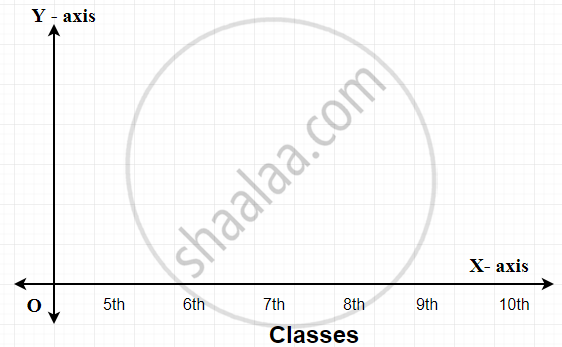3. Choose a scale for the Y-axis. For example, 1 unit = 10 girls/boys. Mark the numbers of boys and girls on the Y-axis.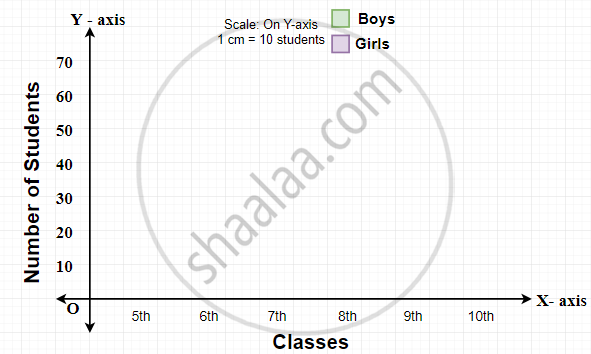4. Using the scale, work out the height of columns required to show the numbers of boys and girls in each class. Use different colours to show the different bars in each set.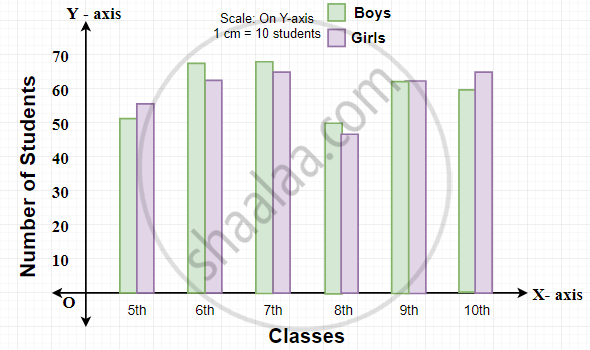2) A mathematics teacher wants to see, whether the new technique of teaching she applied after a quarterly test was effective or not. She takes the scores of the 5 weakest children in the quarterly test (out of 25) and in the half-yearly test (out of 25):

 Students Ashish Arun Kavish Maya Rita Quarterly 10 15 12 20 9 Half-yearly 15 18 16 21 15

To represent this data in the form of a double bar diagram, here are the steps.

1. On a graph paper, draw the X-axis and Y-axis and their point of intersection.
2. Keeping the distance between two sets of joint bars equal shows the students on X-axis.
3. Choose a scale for the Y-axis. For example, 1 unit = 5 marks. Mark the numbers of marks on the Y-axis.
4. Using the scale, work out the height of columns required to show the marks of quarterly and half-yearly of each student. Use different colours to show the different bars in each set.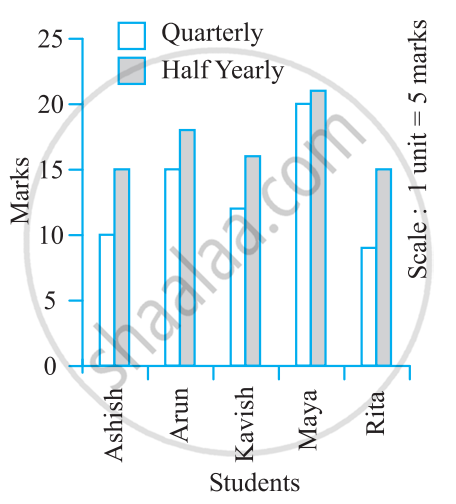If you would like to contribute notes or other learning material, please submit them using the button below.

### Shaalaa.com

Drawing a Double Bar Graph [00:05:07]
S
0%